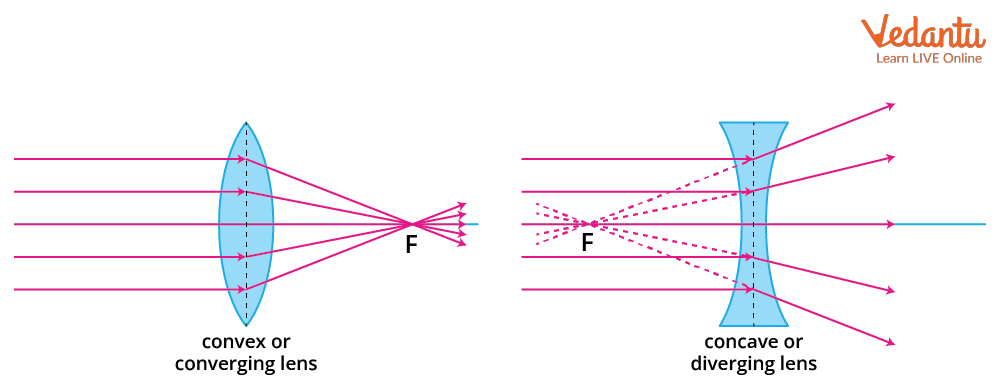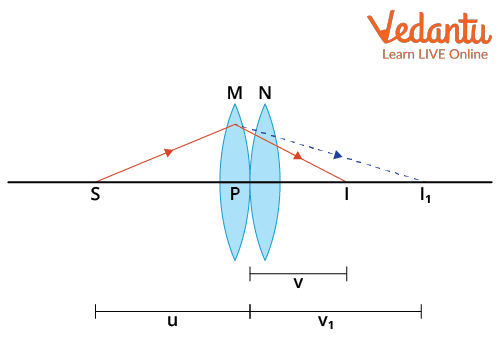Courses
Courses for Kids
Free study material
Free LIVE classes
More

# Combination of Thin Lenses in Contact for JEELIVE
Join Vedantu’s FREE Mastercalss

## What are Lenses?

The word “lens” comes from the Latin word for “lentil,” owing to the similarity in shape between the converging lens and the legume. A lens is a transparent piece of material that has been formed to bend light rays in a particular direction as they pass through it, whether that means having the rays converge at a particular point or diverge as if from a particular point.

The lens's form dictates whether it causes light rays to converge or diverge, and the substance used could be a piece of glass or plastic. Since the lens material has a different index of refraction than the surrounding air, the actual bending of light rays caused by a lens happens because of this. Snell's law of refraction, which connects the difference in angle between the incident and refracted light rays to the indices of refraction for the two materials, describes this behaviour.

We can also say that a lens is created by a transparent material that is bound by two surfaces, either of which is spherical. This indicates that at least one spherical surface confines a lens. The other surface would be planar if there were only one spherical surface. There are two types of lenses depending on the type of spherical surface used, it could either be bulging outwards or converging inwards. The two types of lenses are convex and concave lenses. We’ll see this in more detail.

## Types of Lenses

As previously mentioned before, lenses can be divided into two types. Lenes could be convex or concave.

It's possible for a lens to have two spherical surfaces that protrude. A double convex lens is the name for such a lens. It is referred to as a convex lens. Compared to the edges, the centre is thicker. Light rays converge through a convex lens. Thus, converging lenses refer to convex lenses.

Similarly, a double concave lens is surrounded by two inwardly curved spherical surfaces. The margins are thicker than the centre. Light beams are diverted by such lenses. Diverging lenses are what these are known as. Concave lens is the common name for a double concave lens.Convex And Concave Lens

It is important to remember that the spherical surfaces of the lens form a part of a sphere.

There are some important concepts that have to be known about lenses. We’ll briefly talk about them.

• Focal Length

The focal length of a lens or mirror refers to the separation between its convex or concave surface and its focal point. It is the location where two parallel light beams collide or merge. The focal length of a convex mirror is positive, whereas the focal length of a concave mirror is negative.

• Focal Point

The focal point of a lens is the point where all the parallel rays converge when they pass through the lens.

• Principal Axis

The primary axis is a hypothetical line that connects the centres of the two spheres that make up the spherical lens.

## Combination of Thin Lenses in Contact

Now, we’ll be looking at a combination of thin lenses in contact. A combination of lenses is also known as compound lens. In contrast to compound lens systems, which allow the employment of many lenses along a single common axis, simple lens systems only employ one lens. Two thin lenses kept in touch with one another make up the simplest of these compound lens systems.

Suppose we have two lenses, M and N, with f1 and f2 focal lengths, respectively. S is some object positioned on the common principal axis. The first lens M produces an image at I1. This image at I1 becomes the object of the second lens N. The final image is created at I as we can see from the diagram below. This system of lenses is known as a combination of lenses.Combination of Lenses Diagram

From the diagram, we can see that the object distance from first lens M is u and the image formed by the first lens is at I1 which is at distance v1 from it. So using the lens formula we can write,

$\dfrac{1}{v_{1}}-\dfrac{1}{u}=\dfrac{1}{f_{1}}$

Similarly, for the lens N, the object which is the image formed at I1 is at a distance of v1 from the lens and the final image which is formed at I is at a distance v from it. Again, using the lens formula, we can write

$\dfrac{1}{v}-\dfrac{1}{v_{1}}=\dfrac{1}{f_{2}}$

Now if we add both of these equations from lens M and N,  we get

\begin{align}&\left(\dfrac{1}{v_{1}}-\dfrac{1}{u}\right)+\left(\dfrac{1}{v}-\dfrac{1}{v_{1}}\right)=\dfrac{1}{f_{1}}+\dfrac{1}{f_{2}} \\ &\dfrac{1}{v}-\dfrac{1}{u}=\dfrac{1}{f_{1}}+\dfrac{1}{f_{2}} \end{align}

Now if we notice carefully, from the above equation we can replace the term $\dfrac{1}{f_{1}}+\dfrac{1}{f_{2}}=\dfrac{1}{F}$

This means that the combination of lenses is replaced by a single lens having a focal length F and it forms the image at I when the object is placed at S. F is the focal length of the combination of lenses.

The final equation of the combination of these lenses will hence be

$\dfrac{1}{v}-\dfrac{1}{u}=\dfrac{1}{F}$

Now, we know that the power of a lens is the reciprocal of its focal length. So, the power of the combination will be the sum of the individual powers of the lenses. If we consider the power of first lens to be P1 and the power of the second lens to be P2, then the power of the combination will be,

P=P1+P2

We just considered two lenses, but if we had n lenses having focal lengths f1, f2,f3,.....fn respectively then the focal length of their combination would be

$\dfrac{1}{F}=\dfrac{1}{f_{1}}+\dfrac{1}{f_{2}}+\dfrac{1}{f_{3}}+\ldots+\dfrac{1}{f_{n}}$

This is the combination of lenses formula.

## Conclusion

Lenses are created by a transparent material that is bound by two surfaces, either of which is spherical. There can be two types of surfaces, one that’s bulging outwards and one that’s bending inwards. This makes two lenses which are the convex and concave lenses. Concave lens is called diverging lens and convex lens is known as converging lens. The equivalent focal length F of two lenses kept in contact having focal lengths f1 and f2 is

$\dfrac{1}{f_{1}}+\dfrac{1}{f_{2}}=\dfrac{1}{F}$

For a combination of n lenses having focal lengths f1, f2,f3,.....fn , the equivalent focal length is

$\dfrac{1}{F}=\dfrac{1}{f_{1}}+\dfrac{1}{f_{2}}+\dfrac{1}{f_{3}}+\ldots+\dfrac{1}{f_{n}}$

Last updated date: 26th Sep 2023
Total views: 128.4k
Views today: 3.28k

## FAQs on Combination of Thin Lenses in Contact for JEE

1. What are thin lenses and their uses?

A lens is a piece of glass with two spherical borders and a finite thickness. We refer to a lens as being thin if its thickness is significantly less than its diameter. Two types of thin lenses can be found, which are the convex and concave lenses. Thin lenses find many uses. They are utilised in magnifying glasses, cameras, refracting telescopes, binoculars, eyeglasses, contact lenses, and microscopes. The most important applications of thin lenses are in the eye, the telescope and the microscope.

2. What is the weightage of Combination of Thin Lenses in Contact in JEE ?

Combination of Thin Lenses in Contact comes under the topic of Optics in the JEE Syllabus. This topic forms about 10% of the entire JEE syllabus and every year about 2-3 questions are asked from this particular topic. The chances of the question being asked from the combination of thin lenses in contact is quite high because of the variety of problems that can be framed from this topic. So, this topic should be practised a lot and concepts should be understood thoroughly.